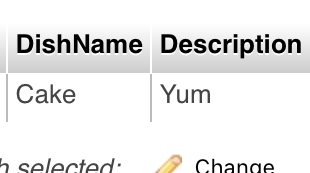# ios 导入mysql数据库,如何将数据从iOS应用程序发布到MySQL数据库？I saw a similar post to my question but his solution did not work for me for some odd reason and it is making me age faster than Obama.

Basically I want to post data from an iOS app to a MySQL database.

iOS code

NSString *strURL = [NSString stringWithFormat:@"http://www.example.com/phpfile.php?dishname=%@&description=%@",textfieldTwo.text, textfieldTwo.text];

NSData *data = [NSData dataWithContentsOfURL:[NSURL URLWithString:strURL]];

NSString *strResults = [[NSString alloc]initWithData:data encoding:NSUTF8StringEncoding];

NSLog(@"%@", strResults);

PHP code

$servername="localhost";$username="admin";

$password="admin";$dbname="dbname";

$conn=mysqli_connect($servername, $username,$password, $dbname); if (!$conn)

{

die("Connection failed: " . mysqli_connect_error());

}

$dishname=$_POST['dishname'];

$description=$_POST['description'];

echo "Name : " . $dishname; //echo "Mail : ".$mail;

$sql="insert into RecipeFeed (DishName, Description) values ('" .$dishname . "', '" . $description . "')";$result=mysqli_query($conn,$sql);

?>

database imageI have no idea whatsoever to why I am having this problem. Any help will be appreciated, thank you!

I figured it out and here is the code below.

PHP

// Create connection

$servername = "localhost";$username = "admin";

$password = "admin";$dbname = "db";

$con=mysqli_connect("localhost","admin","admin","db"); if (!$con) {

die("Connection failed: " . mysqli_connect_error());

echo "Nothing happened";

}else{

}

if (isset ($_GET["firstname"]))$firstname = $_GET["firstname"]; else$firstname = "Null";

if (isset ($_GET["lastname"]))$lastname = $_GET["lastname"]; else$lastname = "Null";

if (isset ($_GET["email"]))$email = $_GET["email"]; else$email = "Null";

if (isset ($_GET["password"]))$password = $_GET["password"]; else$password = "Null";

if (isset ($_GET["timestamp"]))$timestamp = $_GET["timestamp"]; else$timestamp = "Null";

$id = "null"; echo "FirstName : ".$firstname;

echo "LastName : ". $lastname;$sql = "insert into Users (FirstName, ID, LastName, Email, Password, TimeStamp) values ('".$firstname."', '".$id."', '".$lastname."', '".$email."', '".$password."', '".$timestamp."')";

$result = mysqli_query($con, \$sql);

?>

iOS

NSData *dataURL = [NSData dataWithContentsOfURL:[NSURL URLWithString:strURL]];

NSString *strResult = [[NSString alloc] initWithData:dataURL encoding:NSUTF8StringEncoding];

NSLog(@"%@", strResult);01-031万+
11-011115
06-115014
02-108364
09-192796
12-133112
08-211万+
08-147480
04-182896
10-08716点击重新获取扫码支付余额充值